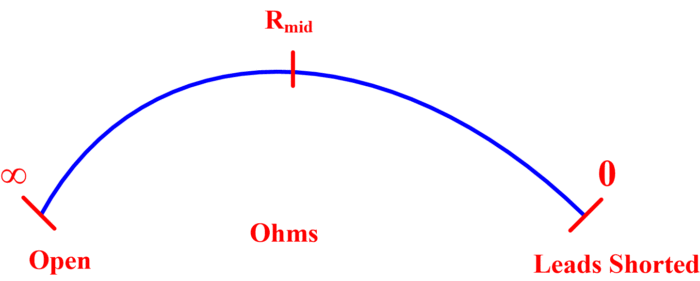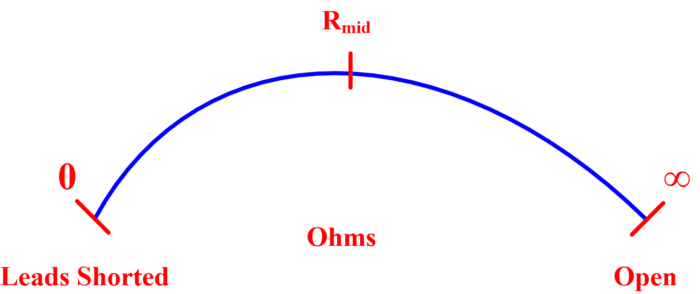# Ohmmeter Basic Concepts and Working Principle

Want create site? Find Free WordPress Themes and plugins.

## Ohmmeter Definition

An ohmmeter is an instrument used to measure the resistance. It is an instrument containing a voltage source and a meter directly calibrated in ohms.

## Series Ohmmeter

One type of ohmmeter is the series ohmmeter, so called because the meter movement is in series with the source of emf and the unknown resistance. The circuit diagram and basic ohmmeter scale are shown in figure 1.(a)(b)

Fig.1: (a) Circuit; (b) Basic Scale

If the terminals of the ohmmeter are left open, ${{R}_{x}}=\infty$ and no current flows. As shown on the basic scale, zero meter deflection corresponds to   ${{R}_{x}}=\infty$. However, when the terminals or leads are shorted, ${{R}_{x}}=0$ and maximum current flows. So that exactly full-scale current flows when  ${{R}_{x}}=0$ , a zero adjust control is provided. The zero adjust allows one to calibrate the ohmmeter with the test leads shorted, thus compensating for lead resistance and battery aging.

If the sum of all the resistances internal to the ohmmeter is called ${{R}_{i}}$,

${{R}_{i}}={{R}_{1}}+{{R}_{2}}+{{R}_{m}}\text{ (1)}$

And the meter current for any value of ${{R}_{x}}$ is

$I=\frac{E}{{{R}_{i}}+{{R}_{x}}}\text{ (2)}$

It follows from equation (2) that if full-scale current flows when${{R}_{x}}=0\text{ }\Omega$, half scale current flows when  ${{R}_{x}}={{R}_{i}}$ . Within the limitations of the emf and meter mechanism used, the internal resistances R1 and R2 are selected to provide a particular mid-scale resistance, Rmid.

Notice that for the left half of the ohmmeter scale, resistances between $\infty \text{ and }{{\text{R}}_{\text{mid}}}$  are indicated; for right half, resistances between ${{\text{R}}_{\text{mid}}}\text{ and 0}$ are indicated. The resulting scale is nonlinear, and at either scale end, the accuracy is poor. Therefore, multi-range ohmmeters having different mid-scale resistances values are desirable. The different scales are obtained through the use of range switching, meter mechanism shunts, and different potential sources.

## Shunt Ohmmeter

The second type of ohmmeter is the shunt ohmmeter, so called because the meter movement is in parallel with the unknown resistance. The basic shunt ohmmeter circuit and scale are shown in figure 2.(a)(b)

Fig.2: The Shunt Ohmmeter: (a) Circuit (b) Basic Scale

Notice that a switch, S, is necessary to prevent current flow from the source of emf when the ohmmeter is not in use.

If the terminals of the shunt ohmmeter are shorted, ${{R}_{x}}=0\text{ }\Omega$ and all current is shunted away from the meter mechanism. However, when the terminals are open, ${{R}_{x}}=\infty$ and maximum current flows. As before, a control is provided for the adjustment of full-scale deflection, but it is now an infinity adjust (∞ adjust). As in the series-type ohmmeter, when the unknown resistance equals the meter resistance, the meter reading is at half scale. In comparison to the series type, though, the shunt ohmmeter has a low meter resistance, making it particularly useful for unknown resistances that are relatively low. Regardless of the type of ohmmeter used, one must be certain that it is not connected to an energized or active circuit.

Did you find apk for android? You can find new Free Android Games and apps.